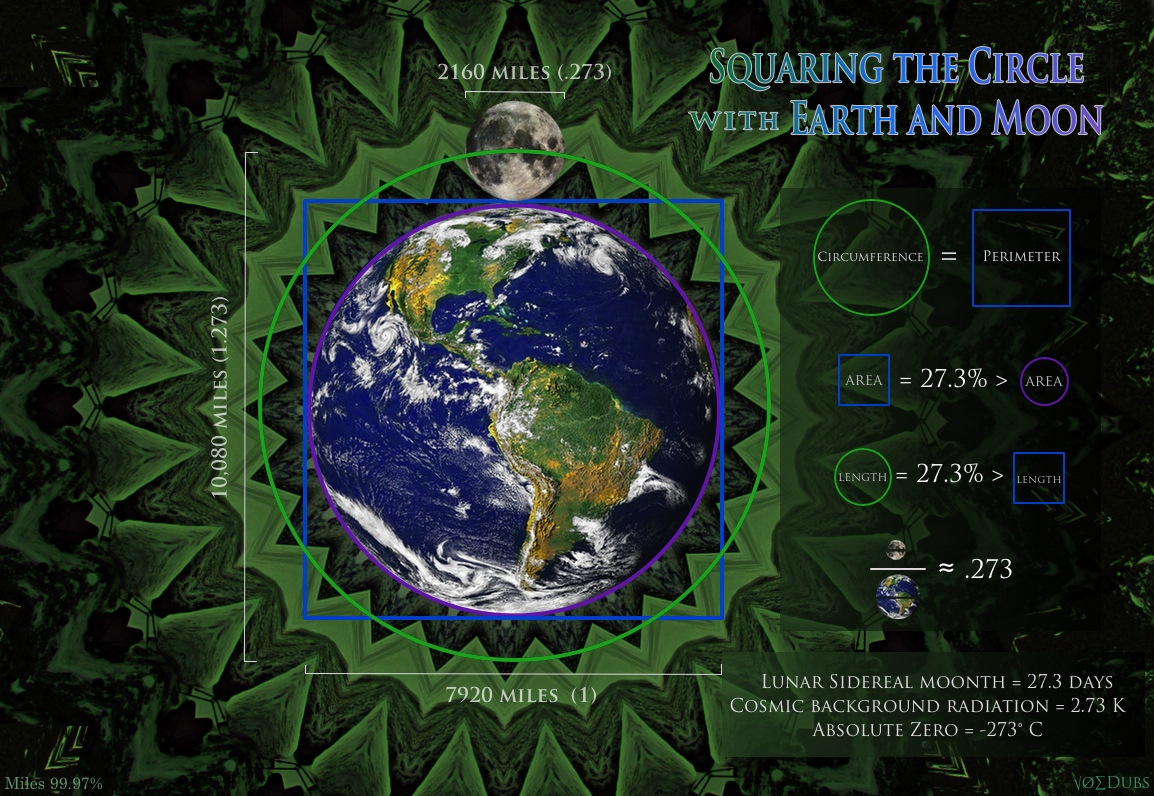## Square the Circle with Earth and MoonWhen we compare the size of Earth and Moon strange geometric synchronicities appear. The most fascinating of all is the ancient philosophical concept of ‘squaring the circle‘, that is drawing a square with the same area as that of the circle. You can also ‘square the circle’ with equal perimeters, which is what the Earth-Moon system do, to a very high degree of accuracy (99.97%). The Earth and Moon’s diameters can be described as a simple ratio, 11:3, when comparing one to another. It turns out that this ratio is the solution to ‘squaring the circle’ (of equal perimeter).   The Moon describes a circle that has the same circumference as the square’s perimeter that surrounds Earth. This fact was discovered or rediscovered by the late and great John Michell. The magic number found in these geometries is 273, or more specifically 2732.  I…

6

## Synergistic Mathemagics in the Solar SystemThe way we measure space and time is based on a synergistic and mathemagical canon of number that’s intrinsic to our solar system as well as the proportions of man.  As above so below. It just so happens that the Moon’s diameter is almost exactly 2160 miles (99.99+%), the same amount of degrees on the surface of a cube.  Earth’s circumference at the equator is almost exactly 21,600 nautical miles. (99.9+%) The Earth and Moon ‘square the circle‘ Square the Circle: The Moon is the perfect size to solve the riddle. It’s huge in fact, larger than any other in proportion to its planet. Some say we have a double planet system. The Moon is 27.3% the size of Earth. It also happens to take 27.3 days to complete an orbit around Earth. Need I point out the fact that the Moon influences our emotions. Women’s menstruation cycles last…

4